Functional Analysis.

The Group for Functional Analysis will conduct research primarily in Operator Theory, Operator Algebras, Harmonic Analysis and Function Spaces, Riemann-Hilbert Problems, and their applications to Physics, Engineering and other areas of Mathematics. Six research lines to pursue the goals of the group will be explored.

Operator Algebras and Index Theory
• C*-crossed products of operator algebras. Generalizations of the local trajectory method on orbits.
• Symbolic calculus for non local C*-algebras of singular operators and for C*-algebras generated by a finite number of projections onto spaces of polyanalitic and polyharmonic functions.
• C*-algebras generated by partial isometries on domains which admit exact Dzhuraev formulas.
• Fredholm index theory for classes of pseudo differential operators in particular using Lie groupoid techniques.
• Algebras of operator sequences, such as algebras generated by variable Toeplitz matrices, in order to obtain conditions for stability.
Harmonic Analysis and Function Spaces
• Mapping properties of operators of Harmonic Analysis such as Hardy, maximal, singular, potential, hypersingular operators, in new function spaces in particular variable exponent generalized Morrey-Campanato spaces and Herz-type spaces.
• New variable exponent function spaces of Adam-Herz type and their Grand versions. The structure and the action of operators of Harmonic Analysis.
• Weighted estimations of operators of Harmonic Analysis in new functions spaces and characterization of admissible weights.
Riemann-Hilbert Problems and Analytic Functional Spaces
• AP factorisation of triangular matrix function related to convolution type operators using in particular the corona theorem.
• Factorisation on Riemann surfaces of matrix functions that belong to classes of loop groups.
• Definition of removal boundary in spaces of polyanalytic functions and properties of several spaces of polyanalytic functions depend on the Cantor-Bendixson rank of the boundary.
• Applications of polyanalytic analysis to the areas of signal analysis, quantum physics and random matrices.
Convolution Type Operators and Singular Integral Operators with Shifts
• Convolution type operators on locally bounded spaces. Factorization for decomposing complete locally bounded function algebras.
• Fredholm criterion and the corresponding index formula for singular integral operators with a backward shift on Lebesque spaces.
Application of Operator Theory to Mathematical Physics
• Explicit solutions of canonical boundary values and interface problems in diffraction theory. Operator factorization and operator relations.
• Special classes of pseudodifferential and Fourier integral operators in the context of boundary value problems on conical Riemann surface.
• Applications of Riemann-Hilbert problems to the study of integrable systems both continuous and discrete. Explicit solutions to the equations defining integrable systems.
Applications to Numerical Analysis and Computational Algorithms
• Design and implementation of computational applications using the computer algebra system Mathematica, allowing the study and development of some topics of Operator Theory. Calculation techniques for computing singular integrals, algorithms for solving integral equations and factorization of matrix functions.
• Numerical stability for equations involving Toeplitz plus Hankel operators and asymptotic behavior of the condition number, spectrum and pseudospectrum.

Close

News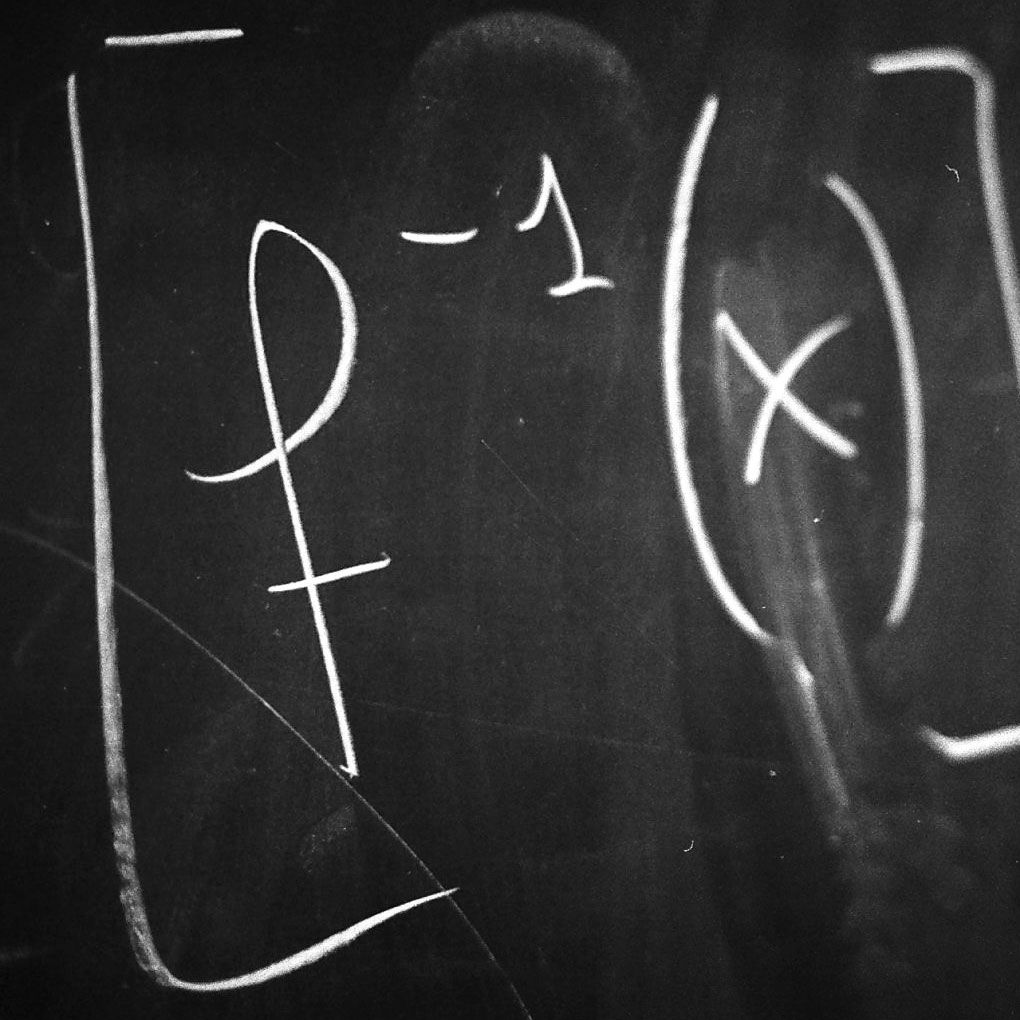IWOTA 2019

Published Jan 30, 2019 in FA LACS

The 30th International Workshop on Operator Theory and its Applications, IWOTA 2019 will be held from Monday July 22nd through Friday July 26th, at Instituto Superior Técnico, University of Lisbon, Portugal. IWOTA 2019 will be focused on the latest developments in Functional Analysis, Operator Theory and related fields. IWOTA 2019 intends to bring together mathematicians and engineers […]

See all News

Members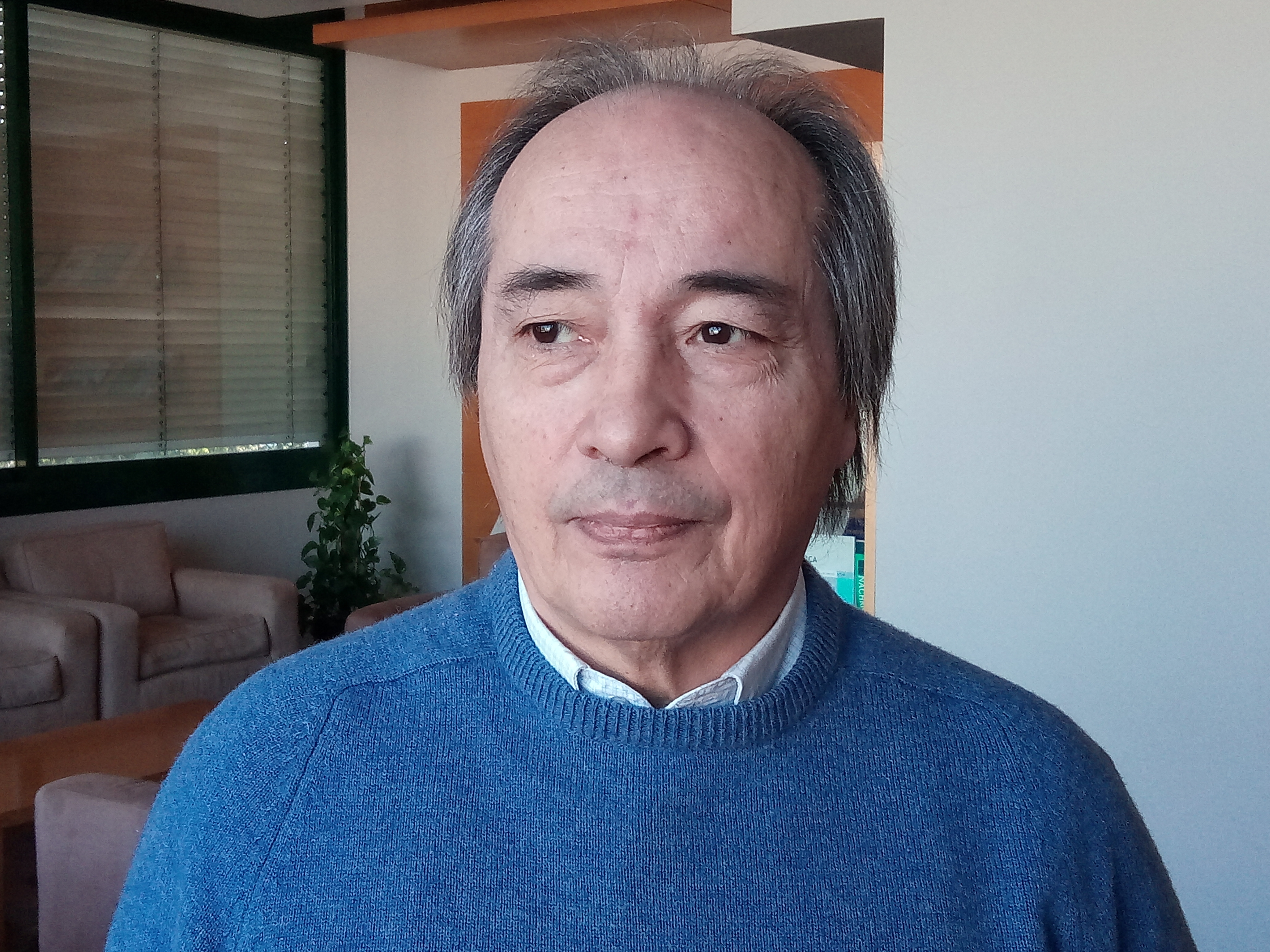Amarino B. Lebre

Instituto Superior Técnico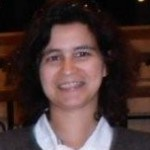Ana Conceição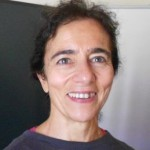Ana Moura Santos

Instituto Superior Técnico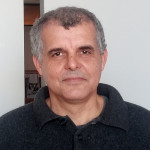António Bravo

Instituto Superior Técnico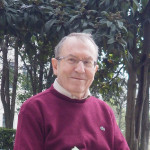António F. dos Santos

Instituto Superior Técnico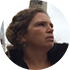Catarina C. Carvalho

Instituto Superior Técnico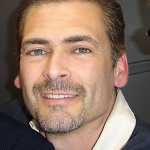Cláudio Fernandes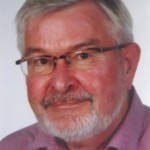Frank Speck

Instituto Superior TécnicoHelena Mascarenhas

Instituto Superior TécnicoJuan Rodríguez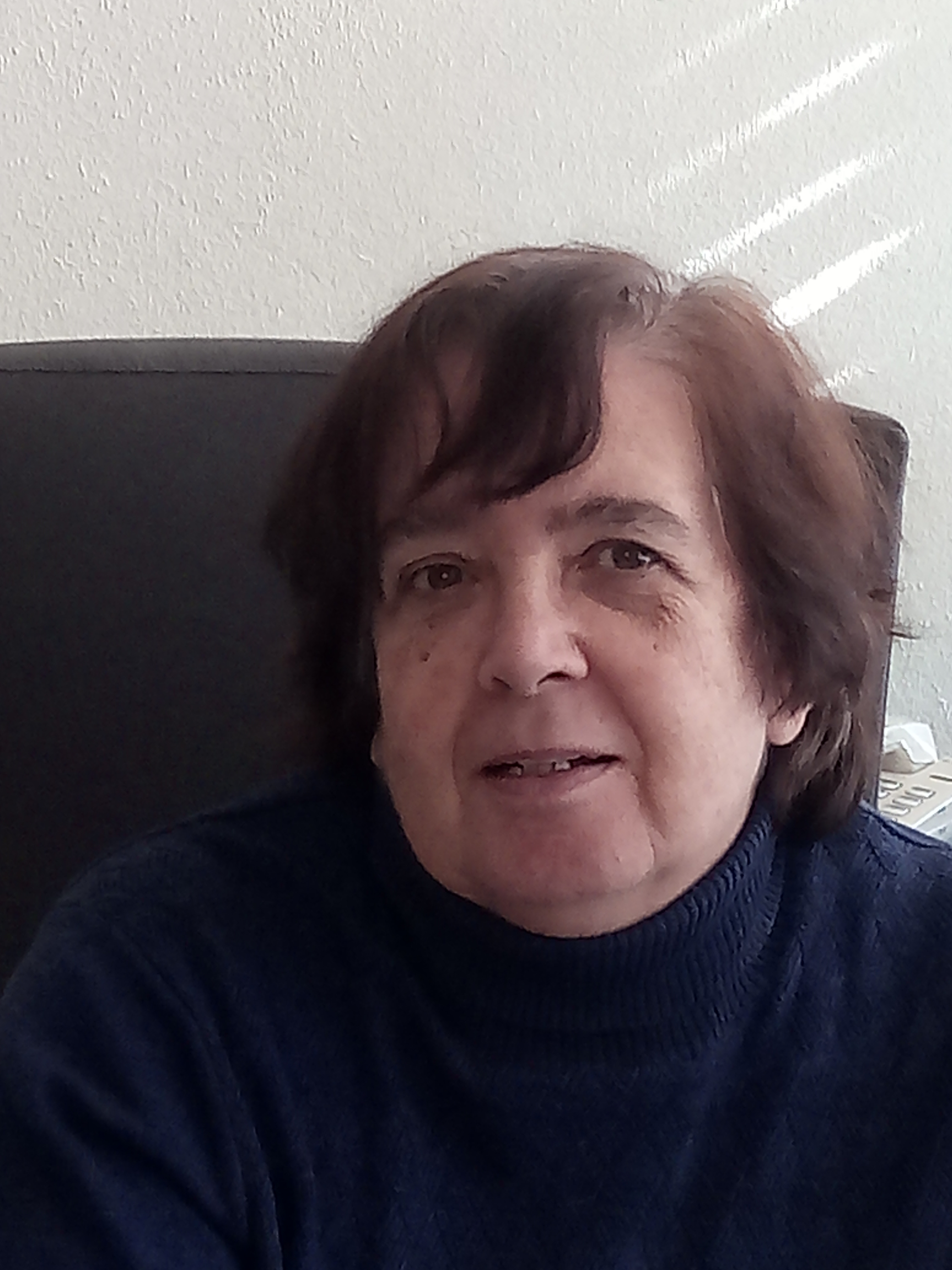M. Amélia Bastos

Instituto Superior Técnico

PresidentNuno Cirilo António

Instituto Superior Técnico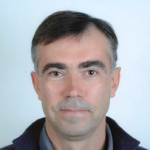Oleksiy KarlovichPaulo Lopes

Instituto Superior Técnico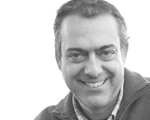Pedro A. Santos

Instituto Superior Técnico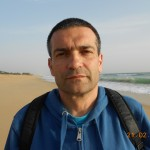Rui Marreiros